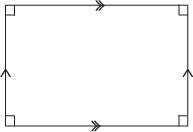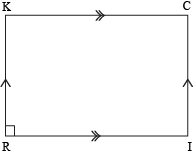Study Guide

## Rectangles

You've probably known what a rectangle was since you were in preschool. We have to admit, they're pretty popular shapes. In the quadrilateral yearbook, rectangles would be voted best dressed, while parallelograms would be voted most likely to get stuffed in a locker. Poor guys.

Until now, you've probably never thought of rectangles as being like parallelograms, but the truth is that the two of them are only about as different as night and...later that night. In fact, just like a rhombus, a rectangle is a special type of parallelogram.

A rectangle is a parallelogram with four right angles. This also means that if we have a parallelogram with four right angles, we really have a rectangle.Of course, all it takes is one right angle to turn a parallelogram into a rectangle. Don't you believe us?

### Sample Problem

Given: RICK is a parallelogram, ∠R is a right angle.
Prove: RICK is a rectangle.

First, we need to draw a rectangle according to our definition.Statements Reasons 1. RICK is a parallelogram Given 2. ∠R is a right angle Given 3. ∠R ≅ ∠C and ∠I ≅ ∠K Opposite angles of a parallelogram are congruent 4. m∠R = 90° Definition of right angle (2) 5. m∠C = 90° Definition of angle congruence (3, 4) 6. ∠R and ∠I are supplementary Consecutive angles of parallelograms are supplementary (1) 7. m∠R + m∠I = 180° Definition of supplementary angles (6) 8. m∠I = 90° Substitution, algebra (4, 7) 9. m∠K = 90° Definition of angle congruence (3, 8) 10. ∠I, ∠C, and ∠K are right angles Definition of right angle (5, 8, 9) 11. RICK is a rectangle Definition of a rectangle (1, 2, 10)

It turns out that there's more to rectangles than meets the eye. Let's hope they won't be meeting anyone's eye at all, though—those vertices can be pretty sharp.

We've talked about the diagonals of parallelograms (check: they bisect each other) and the diagonals of rhombi (check: they're perpendicular), but what about the diagonals of rectangles? What do they have to say for themselves? Whatever it is, they'd probably say the same exact thing. Hint, hint.

That's right. The two diagonals of a rectangle are congruent to one another. One hundred percent, completely, absolutely, for reals congruent. Of course, that's in addition to the fact that they bisect each other (or have you already forgotten that a rectangle is just another type of parallelogram?).

Now that we know what rectangles are, we need to know how to identify them. Unfortunately, not all of 'em wear name tags, and we don't want to be those people at the Parallelogram Party who can't pick out a rectangle to save their lives. Yikes. How embarrassing.

So, if we meet a quadrilateral at the Parallelogram Party without a name tag, how do we know if it's a rectangle? We can't ask it—that'd be rude. So we have three easy ways to tell:

1. If a parallelogram has one right angle, it's a rectangle.
2. If a parallelogram has congruent diagonals, it's a rectangle.
3. If a quadrilateral is equiangular, it's a rectangle.

Wait a second. The first two ways specify that we need to be dealing with a parallelogram first and foremost, but the third talks about any quadrilateral. Is that right? We might want to double-check that, just in case. After all, you wouldn't want to be labeled something you aren't, so let's make sure that these quadrilaterals really are rectangles.

### Sample Problem

Given: LISA is an equiangular quadrilateral.
Prove: LISA is a rectangle.

 Statements Reasons 1. LISA is an equiangular quadrilateral Given 2. ∠L ≅ ∠I ≅ ∠S ≅ ∠A Definition of equiangular (1) 3. m∠L = m∠I = m∠S = m∠A Definition of congruent angles (2) 4. m∠L + m∠I + m∠S + m∠A = 360° The internal angles of a quadrilateral add up to 360° (1) 5. 4(m∠L) = 360° Substitution (3, 4) 6. m∠L = 90° Division property of equality (5) 7. m∠L = m∠I = m∠S = m∠A = 90° Transitive property (3, 6) 8. ∠L, ∠I, ∠S, and ∠A are right angles Definition of a right angle (7) 9. LISA is a rectangle Definition of rectangle (1, 8)

Wonderful! We've proven that LISA is a rectangle simply by being an equiangular quadrilateral. This gives millions of young quadrilaterals out there hope that if they work really hard at the right angle, they can one day become rectangles. Thanks, LISA. You're an inspiration.

Hopefully we can do more than pick rectangles out of a lineup. Knowing about rectangles can open plenty of doors and windows for us. This is especially true if we consider that most doors and windows are rectangular. For instance, we can use our knowledge of rectangles (and parallelograms, right triangles, algebra, and trigonometry) to calculate side lengths, diagonal lengths, and practically anything your heart desires.

### Sample Problem

In rectangle BRAD, BY and AY each measure 5 units. If the dimensions of the rectangle are x – 1 by x + 1, what is the value of x?Because BRAD is a rectangle, we know that BARD. Of course, since BA = 10 units in length, RD = 10 units as well. Easy enough, right?

If we look at one of the diagonals of the rectangle, we can see that we have a right triangle. For instance, ΔRAD is a right triangle with hypotenuse RD of length 10, and legs of lengths x + 1 and x – 1. Why don't we invite our good ol' friend Pythagoras to help us out a little?

a2 + b2 = c2
(x – 1)2 + (x + 1)2 = 102

All we need to do is simplify and solve for x, and we should be good to go.

x2 – 2x + 1 + x2 + 2x + 1 = 100
2x2 + 2 = 100
2x2 = 98
x2 = 49
x = ±7

Uh oh. We've got two options here: +7 or -7. Which one is it? Well, let's think back to what we're talking about. In the diagram, x + 1 and x – 1 are dimensions. They're lengths, and lengths can't be negative. So the dimensions of the rectangle are 6 and 8 units.